# Natural numbers - 5th grade (10y) - math problems

#### Number of problems found: 82

• DigitsWrite the smallest and largest 2-digit natural number.
• Sum of the digitsHow many are two-digit natural numbers that have the sum of the digits 9?
• Primes 2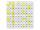Which prime numbers is number 2025 divisible?
• Roman numeralsWrite numbers written in Roman numerals as decimal.
• Written numberPlace+values x ten thousands =30 thousands
• Weeks38 weeks is equal to how many days?
• Integers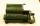May be the sum of two integers less than their difference?
• Quotient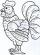Determine the quotient (q) and the remainder (r) from division numbers 100 and 8. Take the test of correctness.
• DivisionWhich number in division 16 give 12 and the rest 3?
• House numbering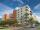The residential house has three entrances numbered even numbers, successive immediately behind. The sum of the two numbers on the outside entrances is 68. Calculate the middle of these three numbers.
• Expression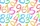If A=2 B=3 evaluate expression A(B+A) and multiply it by A
• What is missing (1000)What number is to add to get 1000?
• Cubic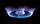Convert 660 m3 of natural gas volume to energy units kWh. Combustion of 1 cubic meter of natural gas is released energy 10.55 kWh.
• Identity123456789 = 100 Use only three plus or minus characters to correct previous identity/equation.
• SavingMom said that Suzan saves about 120 EUR (rounded to tens). How many euros could she at least save (minimum)?
• Equation algebraogram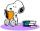Solve the equation: oco + ivo = cita How much has the task of solutions?
• Unknown numberI think the number: Its half is 16 bigger than his quarter. I think the number N . ..
• ClassmatesRoman is ranked 12th highest and eleventh lowest pupil. How many classmates does Roman have?
• The number 3Ski organizers should print the start numbers from 1 to 45. How many times will they use the number 3 when printing?
• Number unknownAdela thought the two-digit number, she added it to its ten times and got 407. What number does she think?

Do you have an interesting mathematical word problem that you can't solve it? Submit a math problem, and we can try to solve it.

We will send a solution to your e-mail address. Solved examples are also published here. Please enter the e-mail correctly and check whether you don't have a full mailbox.

Please do not submit problems from current active competitions such as Mathematical Olympiad, correspondence seminars etc...

Natural numbers - math problems. Examples for 5th grade (the fifth graders).